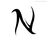### How do I move a record to another table?

How do I move a record from one table to another?

If it is a one time thing and with a limited amount of data, then you can:

1) manually input the data into Table2

or

2) export the record from Table1 and then import the record into Table2

If this is an ongoing task then you can:

1) do step 2 from above

or

2) investigate the create function and possibly the for function.

Thank you. there is no way to directly move a record to another table in the same DB?

Ninox would have to develop some function like the export/import function. There would have to be a screen where it asks you to line up the fields that you want to "move" the record to, then ask if you want to keep/delete the "old" one. I don't know if any DB has this function built in.I'm using this code for this task (copy the record to table B and delete the record from table A)

---

let reply := dialog("", "Would you like to continue moving this record to Table B", ["Yes", "No"]);
let TempB := (create 'Table B');
let myT := Text;
let myN := Number;
TempB.(Text := myT);
TempB.(Number := myN);
delete this;
openRecord(TempB);